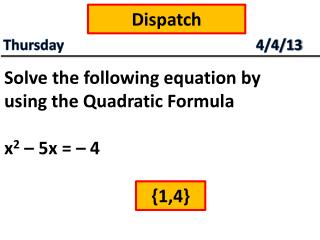DownloadDownload PresentationDispatch

# Dispatch

Télécharger la présentation## Dispatch

- - - - - - - - - - - - - - - - - - - - - - - - - - - E N D - - - - - - - - - - - - - - - - - - - - - - - - - - -
##### Presentation Transcript

1. Dispatch Thursday4/4/13 Solve the following equation by using the Quadratic Formula x2 – 5x = – 4 {1,4}

2. We will be able to determine how many solutions does a given quadratic equation have. Solving Quadratic Equations Standard: 22.0

3. REAL LIFE APPLICATION Mr. Hernandez went bungee jumping on his recent trip to Cabo San Lucas. Before he decided to jump he thought to himself “Hey, I better make sure that I am high enough to not hit the ground when I jump”. Mr. Hernandez, as the great math genius that he is figured out that the elasticity of the bungee chord created the equation h = t2 – 9t + c, where t represents time and c represents his initial height. What should the value of c be so Mr. Hernandez does not hit the ground?

4. ACTIVITY STEP 1: Solve the Quadratic Equation that was assigned to you using the Quadratic Formula in the “My Equation Box” STEP 2: Have your elbow partner check your answer STEP 3: As a group, share your answers to complete “My Group Findings” Chart

5. ACTIVITY STEP 4: Before asking me to check your answers, check with each other. STEP 5: Complete the Questions that follow

6. Making Connections • What relationship (pattern) did you notice between the Quadratic Formula and the number of solutions? (Hint: look at how you solved the problem) • What conclusions can you make about finding the number of solutions in a Quadratic Equation?

7. REAL LIFE APPLICATION Mr. Hernandez went bungee jumping on his recent trip to Cabo San Lucas. Before he decided to jump he thought to himself “Hey, I better make sure that I am high enough to not hit the ground when I jump”. Mr. Hernandez, as the great math genius that he is figured out that the elasticity of the bungee chord created the equation h = t2 – 9t + c, where t represents time and c represents his initial height. What should the value of c be so Mr. Hernandez does not hit the ground? (Hint: Discriminant)